# HBSE 8th Class Maths Solutions Chapter 8 Comparing Quantities InText Questions

Haryana State Board HBSE 8th Class Maths Solutions Chapter 8 Comparing Quantities InText Questions and Answers.

## Haryana Board 8th Class Maths Solutions Chapter 8 Comparing Quantities InText Questions

Try These (Page 119)

Question 1.
In a primary school, the parents were asked about the number of hours they spend per day in helping their children to do homework. There were 90 parent who helped for $$\frac{1}{2}$$ hour to 1$$\frac{1}{2}$$ hours. The distribution of parents according to time for which, they said they helped is given in the adjoining figure; 20% helped for more than 1$$\frac{1}{2}$$ hours per day; 30% helped for $$\frac{1}{2}$$ hour to 1$$\frac{1}{2}$$ hours; 50% did not help at all. It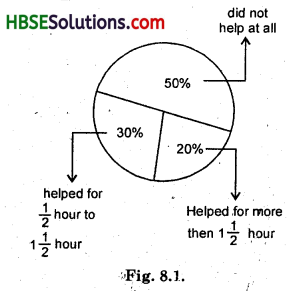Using this answer the following :
(i) How many parents were surveyed ?
(ii) How many said that they did not help ?
(iii) How many said that they helped for more than 1$$\frac{1}{2}$$ hours ?
Solution:
(i) Let total no. of parents be x 30% of x helped for $$\frac{1}{2}$$ hour to 1$$\frac{1}{2}$$ hour
So 30% of x = 90
$$\frac{30}{100}$$ × x = 90 or x = $$\frac{90 \times 100}{30}$$ = 300
Number of parents = 300.

(ii) 50% parents did not help,
So number of parents did not help
= 50% of 300 = $$\frac{50}{100}$$ × 300 = 150

(iii) 20% parents helped for more than 1$$\frac{1}{2}$$ hours
So, number of such parents
= 20% of 300 = $$\frac{20}{100}$$ × 300 = 60

Try These (Page 121)

Question 1.
A shop gives 20% discount. What would the sale price of each of these be ?
(a) A dress marked at Rs. 120.
(b) A pair of shoes marked at Rs. 750.
(c) A bag marked at Rs. 250.
Solution:
(a) 20% discount means On Rs. 100, discount is Rs. 20
On Re. 1 = Rs. $$\frac{20}{100}$$
∴ On 120 = $$\frac{20}{100}$$ × 120 = 24
Sale price = M.P. – Discount
= 120 – 24 = 96
∴ Sale price = Rs. 96

(b) 20% discount means
20% discount on M.P.
⇒ Selling price = 100 – 20
= 80% on M.P.
∴ Sale price of shoe of MP Rs. 750
= 80% of 750
= $$\frac{80}{100}$$ × 750 = 600
∴ Sale price of shoe = Rs. 600.

(c) 20% discount means
Selling price = 80% of M.P.
∴ Selling price of bag of M.P. Rs. 250
= 80% of 250
= $$\frac{80}{100}$$ × 250 = 200
∴ Sale price of bag = Rs. 200.Question 2.
A table marked at Rs. 15,000 is available for Rs. 14,400. Find the discount given and the discount per cent.
Solution:
M.P. = Rs. 15000
S.P. = 1440
Discount = ?
Discount % = ?
Discount = M.P. – S.P.
= 15000 – 14400 = 600
Discount % = $$\frac{Discount}{M.P.}$$ × 100
= $$\frac{600}{15000}$$ × 100 = 4%
Discount – Rs. 600, discount % = 4.

Question 3.
An almirah is sold at Rs. 5,225 after allowing a discount of 5%. Find its marked price.
Solution:
M.P. = ?
S.P. = Rs. 5,225
Discount % = 5 %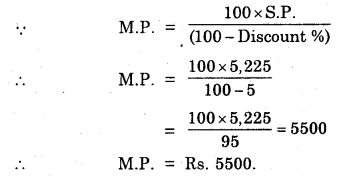Try These (Page 123)

Question 1.
Find selling price (S.P.) if a profit of 5% is made on
(a) a cycle of Rs. 700 with Rs. 50 as overhead charges.
(b) a lawn mower bought at Rs. 1150 with Rs. 50 as transportation charges.
(c) a fan bought for Rs. 560 and expenses of Rs. 40 made on its repairs.
Solution:
(a) Cycle of worth Rs. 700
Overhead charges on cycle = 50
∴ C.P. of cycle = 700 + 50 = 750
Profit % = 5
∴ Profit of Rs. 5 on C.P. of Rs. 100
⇒ S.P. = (100 + 5)% of C.P.
∴ S.P. of cycle of C.P. Rs. 750 = 105% of 750
= $$\frac{105}{100}$$ × 750 = 787.50
∴ S.P. of cycle = Rs. 787.50.

(b) Lawn mower bought in Rs. 1150
Transportation charge = Rs. 50
∴ C.P. of lawn mower = 1150 + 50 = 1200
5% profit on lawn mower is made
∴ S.P. = 105% of C.P.
= $$\frac{105}{100}$$ × 1200 = 1260
∴ Sale price of lawn mower = Rs. 1260.

(c) Fan bought in Rs. 560
Expenses on its repairs = Rs. 40
∴ C.P. of fan = 560 + 40 = 600
5% profit on fan is made
∴ S.P. of fan = 105% of C.P. of fan
= $$\frac{105}{100}$$ × 600 = 630
S.P. of fan = Rs. 630.

Question 2.
A shopkeeper bought two T.V. sets at Rs. 10,000 each. He sold one at a profit of 10% and the other at a loss of 10%. Find whether he made an overall profit or loss.
Solution:
Overall C.P. of each T.V. set = Rs. 10,000
One is sold at a profit of 10%
⇒ If C.P. is Rs. 100, S.P. is Rs. 110
Therefore, when C.P. is Rs. 10,000,
Then, S.P. = Rs. $$\frac{110}{100}$$ × 10000 = 11000
In second case, T.V. at a loss of 10%
⇒ If C.P. is Rs. 100, S.P. is Rs. 90
Therefore, when C.P. is Rs. 10,000
Then, S.P. = Rs. $$\frac{90}{100}$$ × 10000 = 9000
Total C.P. of two sets of T.V.
= Rs. 10000 + Rs. 10000
= Rs. 20,000
Total S.P. of two sets of T.V.
= Rs. 11000 + Rs. 9000
= Rs. 20,000
Since Total S.P. = Total C,P.
⇒ Neither profit nor loss.Try These (Page 124-125)

Question 1.
Find the buying price of each of the following when 5% S.T. is added on the purchase of
(a) A towel at Rs. 50.
(b) Two bars of soap at Rs. 35 each.
(c) 5 kg of flour at Rs. 15 per kg.
Solution:
(a) S.T. of Rs 50 = 50 × $$\frac{10}{100}$$ = Rs. 5
Bill amount = Cost of towel + sales tax
= 50 + 5 = Rs. 55.

(b) S.T. of Rs. 35 = 35 × $$\frac{10}{100}$$ = Rs. 3.50
∴ Bill amount = cost of two bars of soap + S.T.
= 70 + 7 = Rs. 77.

(c) Cost of 5 kg flour = 5 × 15 = Rs. 75
Sales tax = Rs. 75 × $$\frac{10}{100}$$
= Rs. 7.5
Bill amount = Cost of flour + S.T.
= 75 + 7.5
= Rs. 82.50.

Question 2.
If 8% VAT is included in the prices, find the original price of
(a) A TV bought for Rs. 13,500.
(b) A shampoo bottle bought for Rs. 180.
Solution:
(a) 8% VAT mean an article of Rs. 100
will costs Rs. 100 + 8 = Rs. 108
Therefore, when price including VAT is Rs. 108, original price = Rs. 100
∴ When price including VAT is Rs. 13500
Original price = $$\frac{100}{108}$$ × 13500 = 12500
∴ Original price of T.V. = Rs. 12500.

(b) 8% VAT included in the price
⇒ When price including VAT is Rs. 108, original price = Rs. 100
∴ When price including VAT is Rs. 180, original price
= $$\frac{100}{108}$$ × 180 = 166.67
∴ Original price of shampoo = Rs. 166.67.Try These (Page 126)

Question 1.
Find interest and amount to be paid on Rs. 15000 at 5% per annum after 2 years.
Solution:
P = Rs. 15000, R = 5% per annum, T = 2 year, I = ?, A = ?
I = $$\frac{P \times R \times T}{100}$$ × $$\frac{5}{100}$$ × 2 = Rs. 1500
A = P + I
= Rs. 15000 + Rs. 1500
= Rs. 16500.

Try These (Page 129)

Question 1.
Find C.I. on a sum of Rs. 8000 for 2 years at 5% per annum compounded annually.
Solution:
P = Rs. 8000, R = 5% per annum, T = 2 year, C.I. = ?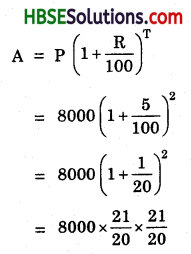= 20 × 21 × 21 = Rs. 8820
C.I. = A – P
= Rs. 8820 – Rs. 8000
= Rs. 820.Try These (Page 130)

Question 1.
Find the time period and rate for each.
1. A sum taken for 1$$\frac{1}{2}$$ years at 8% per annum is compounded half yearly.
2. A sum taken for 2 years at 4% per annum compounded half yearly.
Solution:
1. If the time period yearly and the interest is compounded half yearly, then the time, T will be T × 2
∴ T = 1$$\frac{1}{2}$$ year
= $$\frac{3}{2}$$ × 2 = 3 years.
and, if the rate of interest is R% per annum and the interest is compounded half yearly, then the rate of interest will be $$\frac{R}{2}$$ % per half hear.
R = 8% = $$\frac{R}{2}$$
= 4% per half year.

2. If the time period yearly and the interest is compounded half yearly then the time, T will be T × 2
∴ T = 2 years = 2 × 2 = 4 years
and, if the rate of interest is R% per annum and the interest is compounded half yearly, then the rate of interest will be $$\frac{R}{2}$$ % per half year.
∴ R = 4% = $$\frac{4}{2}$$%
= 2% per half year.

Try These (Page 131)

Question 1.
Find the amount to be paid
(i) At the end of 2 years on Rs. 2,400 at ty5% per annum compounded annually.
(ii) At the end of 1 year on Rs. 1,800 at 8% per annum compounded quarterly.
Solution:
(i) A = ?, T = 2 years, P = Rs. 2400, R = 5% per annum compounded annually.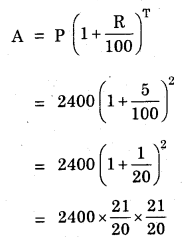= 6 × 441 = Rs. 2646
∴ Amount = Rs. 2646.

(ii) R = 8% per annum compounded quarterly = 2% per quarterly
T = 1 year = 4 quarter
P = Rs. 1800, A = ?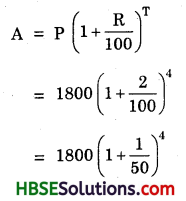= 1800 × $$\frac{51}{50}$$ × $$\frac{51}{50}$$
= Rs. 1872.7
Amount = Rs. 1872.72.Try These (Page 133)

Question 1.
A machinery worth Rs. 10,500 depreciated by 5%. Find its value after one year.
Solution:
P = Rs. 10500, R = 5%, T = 1 year
Cost of machinery after one year
= P(1 – $$\frac{R}{100}$$)1 = 10500(1 – $$\frac{5}{100}$$)1
= 10500(1 – $$\frac{1}{20}$$)1
= 10500 $$\frac{19}{20}$$
= 525 × 19 = Rs. 9975.

Question 2.
Find the population of a city after 2 years, which is at present 12 lakh, if the rate of increase is 4%.
Solution:
T = 2 year, present population, P = 12 lakh
R = 4%, A = ?
= 1200000 (1 + $$\frac{4}{100}$$)2
= 1200000(1 + $$\frac{1}{25}$$)2
= 1200000 × $$\frac{26}{25}$$ × $$\frac{26}{25}$$
= 1297920
∴ Population after 2 years
= 1297920.